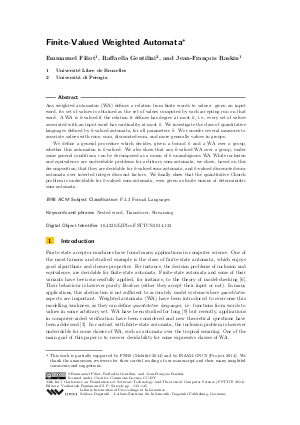Document# Finite-Valued Weighted Automata

### Authors Emmanuel Filiot, Raffaella Gentilini, Jean-Francois Raskin## File

LIPIcs.FSTTCS.2014.133.pdf
• Filesize: 484 kB
• 13 pages

## Cite As

Emmanuel Filiot, Raffaella Gentilini, and Jean-Francois Raskin. Finite-Valued Weighted Automata. In 34th International Conference on Foundation of Software Technology and Theoretical Computer Science (FSTTCS 2014). Leibniz International Proceedings in Informatics (LIPIcs), Volume 29, pp. 133-145, Schloss Dagstuhl - Leibniz-Zentrum für Informatik (2014)
https://doi.org/10.4230/LIPIcs.FSTTCS.2014.133

## Abstract

Any weighted automaton (WA) defines a relation from finite words to values: given an input word, its set of values is obtained as the set of values computed by each accepting run on that word. A WA is k-valued if the relation it defines has degree at most k, i.e., every set of values associated with an input word has cardinality at most k. We investigate the class of quantitative languages defined by k-valued automata, for all parameters k. We consider several measures to associate values with runs: sum, discounted-sum, and more generally values in groups. We define a general procedure which decides, given a bound k and a WA over a group, whether this automaton is k-valued. We also show that any k-valued WA over a group, under some general conditions, can be decomposed as a union of k unambiguous WA. While inclusion and equivalence are undecidable problems for arbitrary sum-automata, we show, based on this decomposition, that they are decidable for k-valued sum-automata, and k-valued discounted sum-automata over inverted integer discount factors. We finally show that the quantitative Church problem is undecidable for k-valued sum-automata, even given as finite unions of deterministic sum-automata.
• Nested word
• Transducer
• Streaming

## Metrics

• Access Statistics
• Total Accesses (updated on a weekly basis)
0